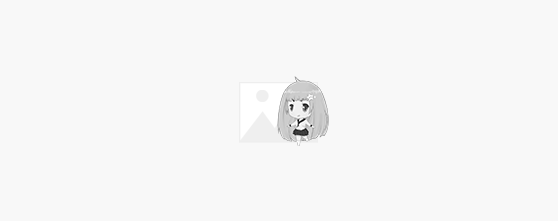# PHP判断输出2-200之间数字是素数的数值

## 代码

```//定义一个功能函数
function is_leap(\$v)
{
\$count = 0; //初始化计数变量\$count
for (\$i = 1; \$i <= \$v; \$i++) {
if (\$v % \$i == 0) {
\$count++;
}
}
if (\$count == 2) { //只有两个可以整除的数,就是素数.
return true;
}
return false;
}
//循环输出2-200之间是素数的值
for (\$i=2; \$i <= 200; \$i++) {
if (is_leap(\$i)) {
echo "\$i ";
}
}```